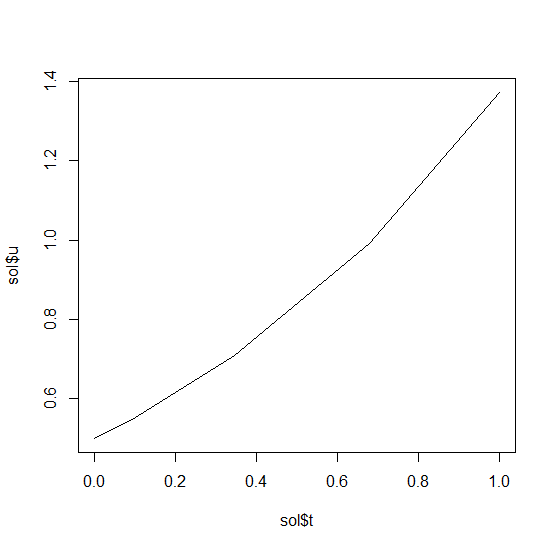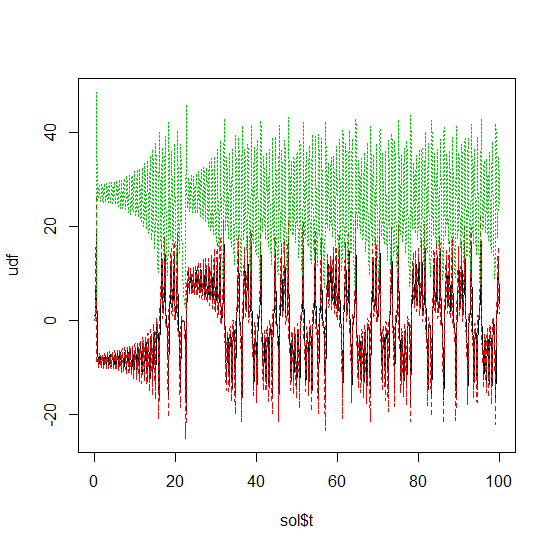# Solving Ordinary Differential Equations (ODE) in R with diffeqr

### 1D Linear ODEs

Let’s solve the linear ODE `u'=1.01u`. First setup the package:

``diffeqr::diffeq_setup()``

Define the derivative function `f(u,p,t)`.

``````f <- function(u,p,t) {
return(1.01*u)
}``````

Then we give it an initial condition and a time span to solve over:

``````u0 = 1/2
tspan <- list(0.0,1.0)``````

With those pieces we call `diffeqr::ode.solve` to solve the ODE:

``sol = diffeqr::ode.solve(f,u0,tspan)``

This gives back a solution object for which `sol\$t` are the time points and `sol\$u` are the values. We can check it by plotting the solution:

``plot(sol\$t,sol\$u,"l")``linear_ode

### Systems of ODEs

Now let’s solve the Lorenz equations. In this case, our initial condition is a vector and our derivative functions takes in the vector to return a vector (note: arbitrary dimensional arrays are allowed). We would define this as:

``````f <- function(u,p,t) {
du1 = p*(u-u)
du2 = u*(p-u) - u
du3 = u*u - p*u
return(c(du1,du2,du3))
}``````

Here we utilized the parameter array `p`. Thus we use `diffeqr::ode.solve` like before, but also pass in parameters this time:

``````u0 = c(1.0,0.0,0.0)
tspan <- list(0.0,100.0)
p = c(10.0,28.0,8/3)
sol = diffeqr::ode.solve(f,u0,tspan,p=p)``````

The returned solution is like before. It is convenient to turn it into a data.frame:

``udf = as.data.frame(sol\$u)``

Now we can use `matplot` to plot the timeseries together:

``matplot(sol\$t,udf,"l",col=1:3)``

Now we can use the Plotly package to draw a phase plot:

``plotly::plot_ly(udf, x = ~V1, y = ~V2, z = ~V3, type = 'scatter3d', mode = 'lines')``timeseries

Plotly is much prettier!

### Option Handling

If we want to have a more accurate solution, we can send `abstol` and `reltol`. Defaults are `1e-6` and `1e-3` respectively. Generally you can think of the digits of accuracy as related to 1 plus the exponent of the relative tolerance, so the default is two digits of accuracy. Absolute tolernace is the accuracy near 0.

In addition, we may want to choose to save at more time points. We do this by giving an array of values to save at as `saveat`. Together, this looks like:

``````abstol = 1e-8
reltol = 1e-8
saveat = 0:10000/100
sol = diffeqr::ode.solve(f,u0,tspan,p=p,abstol=abstol,reltol=reltol,saveat=saveat)
udf = as.data.frame(sol\$u)
plotly::plot_ly(udf, x = ~V1, y = ~V2, z = ~V3, type = 'scatter3d', mode = 'lines')``````